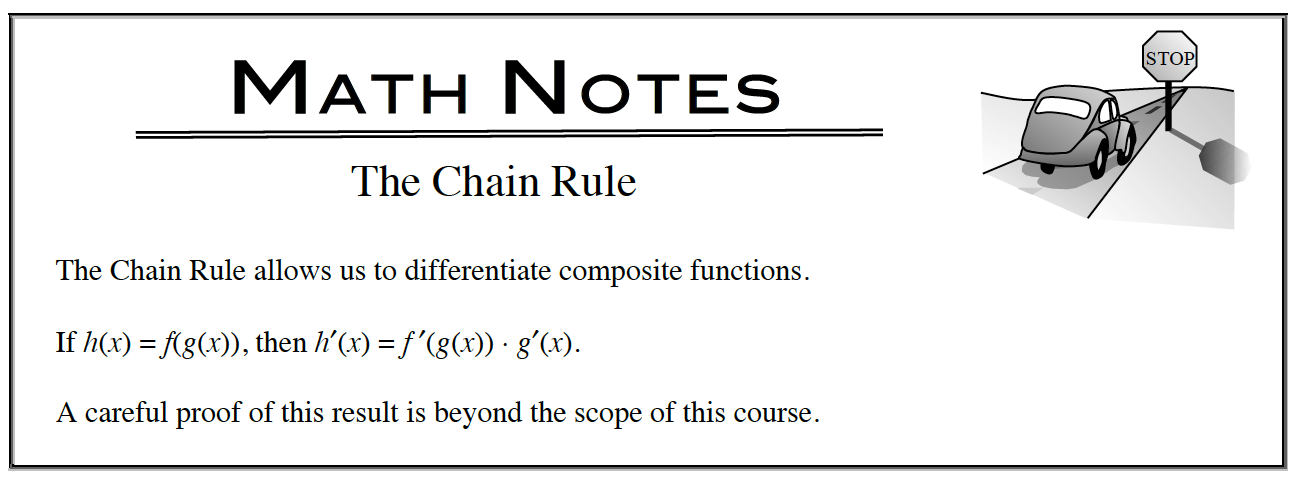### Home > APCALC > Chapter 5 > Lesson 5.2.3 > Problem5-79

5-79.

Sometimes people make mistakes when differentiating using the Chain Rule. Explain the mistakes in the work below. Then write the correct derivatives. Homework Help ✎1. $f^{ }\left(x\right) = –5\left(6x^{4} – 1\right)^{10} + π$
$f^\prime\left(x\right) = –50\left(24x^{3} – 1\right)^{9}$

2. $f^{ }\left(x\right)=\sin\left(x^2-x\right)$
$f^\prime\left(x\right)=−\cos\left(x^2−x\right)$